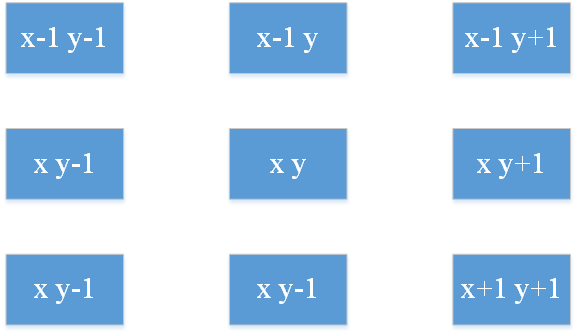# 再来一篇深度优先遍历/搜索总结？

### 思路### 那是怎样得到这样的结果呢？

``````class TreeNode{
int val;
TreeNode left;
TreeNode right;
}
``````

``````class Solution{
public void depthOrderTraversalWithRecusive(TreeNode root){
if(root == null){
return;
}
System.out.print(root.val +"->");
depthOrderTraversalWithRecusive(root.left);
depthOrderTraversalWithRecusive(root.right);
}
}
``````

``````class Solution{
public void depthOrderTraversalWithoutRecusive(TreeNode root){
if(root == null) return;
Stack<TreeNode> stack = new Stack<>();
stack.push(root);
while(!stack.isEmpty()){
TreeNode node = stack.pop();
System.out.print(node.val + "->");
if(node.right != null){
stack.push(node.right);
}
if(node.left != null){
stack.push(node.left);
}
}
}
}
``````

### 扫雷

• 如果一个地雷（`'M'`）被挖出，游戏就结束了- 把它改为` 'X'`
• 如果一个没有相邻地雷的空方块（`'E'`）被挖出，修改它为（`'B'`），并且所有和其相邻的方块都应该被递归地揭露。
• 如果一个至少与一个地雷相邻的空方块（`'E'`）被挖出，修改它为数字（`'1'``'8'`），表示相邻地雷的数量。
• 如果在此次点击中，若无更多方块可被揭露，则返回面板。

``````输入:

[['E', 'E', 'E', 'E', 'E'],
['E', 'E', 'M', 'E', 'E'],
['E', 'E', 'E', 'E', 'E'],
['E', 'E', 'E', 'E', 'E']]

Click : [3,0]

[['B', '1', 'E', '1', 'B'],
['B', '1', 'M', '1', 'B'],
['B', '1', '1', '1', 'B'],
['B', 'B', 'B', 'B', 'B']]
````````````for(int i=-1;i<=1;i++){
for(int j=-1;j<=1;j++){

}
}
``````

• `Click`给出的坐标找出的是地雷，直接返回。
• 否则，进行递归，并标出雷的数量。
``````class Solution{
public char[][] updateBoard(char[][] board,int[] click){
if(board[click][click] == 'M'){
board[click][click] = 'X';
return board;
}
return click(board,click,click);
}
private char[][] click(char[][] board,int x,int y){
int num = getNum(board, x,y);
if(num == 0){
board[x][y] = 'B';
}else{
board[x][y] = Character.forDigit(num,10);
return board;
}
//递归
for(int i=-1;i<=1;i++){
for(int j=-1;j<=1;j++){
if(x + i >= 0 && x + i < board.length&&y + j >=0&&y+j<board.length&&board[x+i][y+j]=='E'){
board = click(board,x+i,y+j);
}
}
}
return board;
}
private int getNum(char[][] board,int x,int y){
int num = 0;
for(int i=-1;i<=1;i++){
for(int j=-1;j<=1;j++){
if(x + i >= 0&&y + j >=0&&x+i<board.length&&y+j<board.length&&board[x+i][y+j]=='M'){
num ++;
}
}
}
return num;
}
}
``````